# Introductory Algebra GlossaryPage 3

#### WATCH ALL SLIDES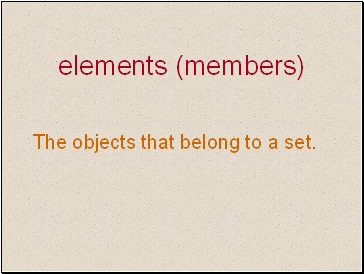## Elements (members)

The objects that belong to a set.

Slide 30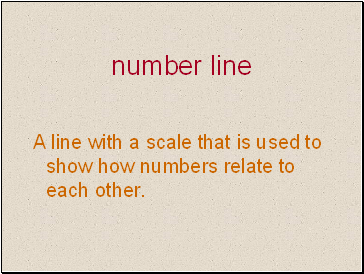## Number line

A line with a scale that is used to show how numbers relate to each other.

Slide 31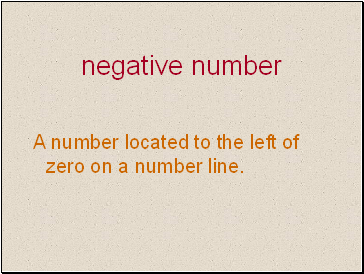## Negative number

A number located to the left of zero on a number line.

Slide 32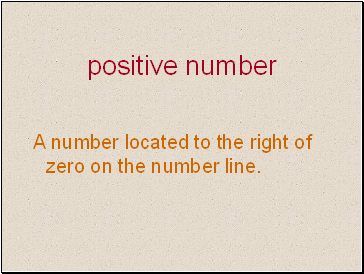## Positive number

A number located to the right of zero on the number line.

Slide 33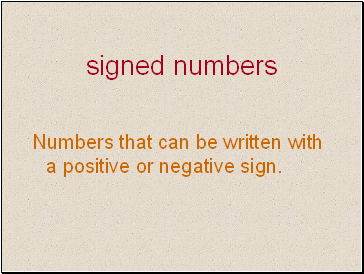## Signed numbers

Numbers that can be written with a positive or negative sign.

Slide 34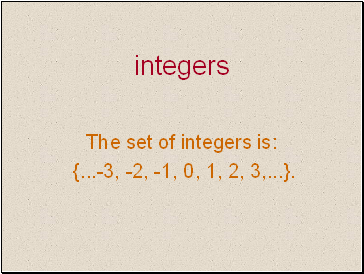## Integers

The set of integers is:

{ .-3, -2, -1, 0, 1, 2, 3, .}.

Slide 35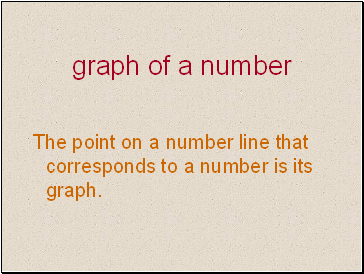## Graph of a number

The point on a number line that corresponds to a number is its graph.

Slide 36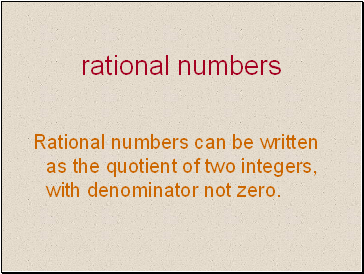## Rational numbers

Rational numbers can be written as the quotient of two integers, with denominator not zero.

Slide 37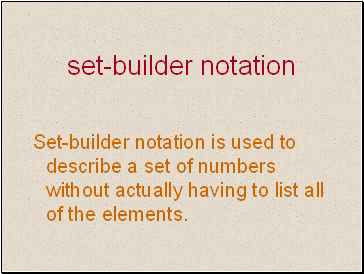## Set-builder notation

Set-builder notation is used to describe a set of numbers without actually having to list all of the elements.

Slide 38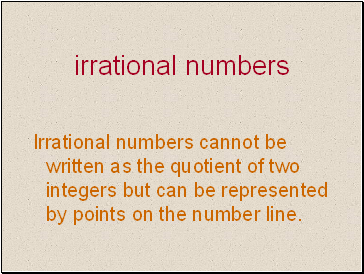## Irrational numbers

Irrational numbers cannot be written as the quotient of two integers but can be represented by points on the number line.

Slide 39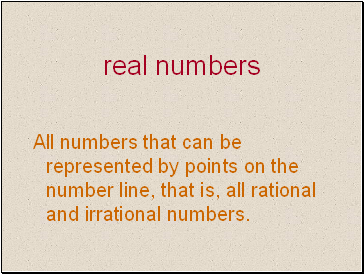## Real numbers

All numbers that can be represented by points on the number line, that is, all rational and irrational numbers.

Slide 40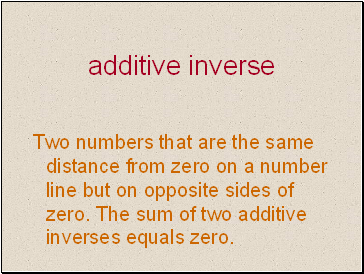Two numbers that are the same distance from zero on a number line but on opposite sides of zero. The sum of two additive inverses equals zero.

Slide 41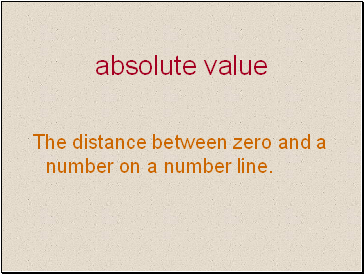## Absolute value

The distance between zero and a number on a number line.

Slide 42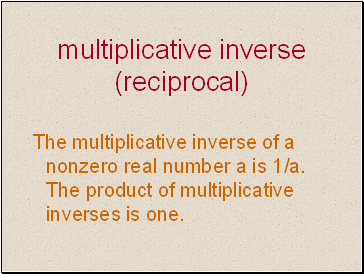## Multiplicative inverse (reciprocal)

The multiplicative inverse of a nonzero real number a is 1/a. The product of multiplicative inverses is one.

Go to page:
1  2  3  4  5